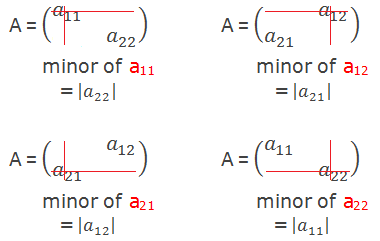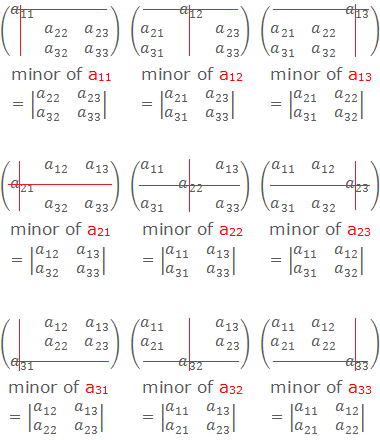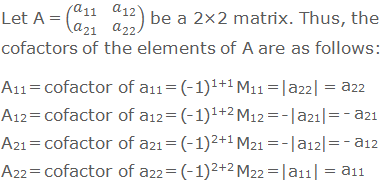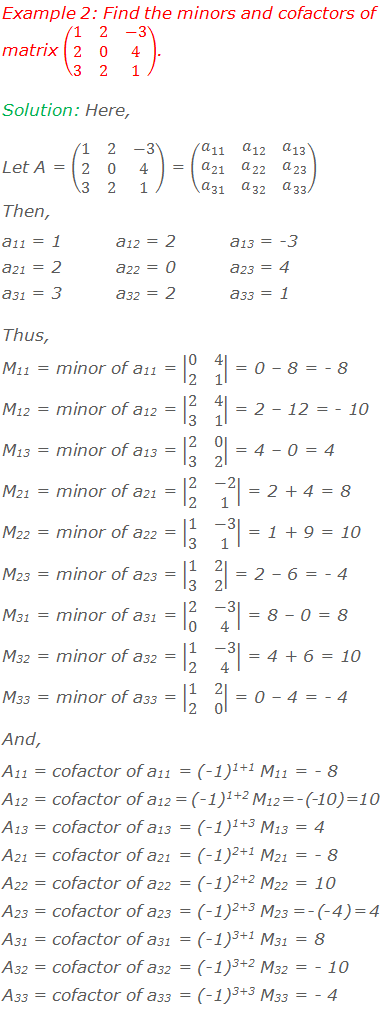# Minors and Cofactors of a Matrix## Minors and Cofactors of a Matrix

### Minors:

Let A be a square matrix and aij is the element in ith row and jth column of A. Then the minor of the element aij is the determinant of the matrix formed by omitting ith row and jth column of A. The minor of element aij is denoted by Mij.********************

10 Math Problems officially announces the release of Quick Math Solver, an Android App on the Google Play Store for students around the world.

********************

#### Minors For 2×2 MatrixLook at the following pattern to find the minors of the elements of a 2×2 matrix:Thus,

M11 = minor of a11 = |a22| = a22

M12 = minor of a12 = |a21| = a21

M21 = minor of a21 = |a12| = a12

M22 = minor of a22 = |a11| = a11

#### Minors For 3×3 MatrixLook at the following pattern to find the minors of the elements of a 3×3 matrix:Thus,### Cofactors:

Let A be a square matrix and aij is the element in ith row and jth column of A. Then the cofactor of the element aij is given by the number (-1)i+j Mij, where Mij is the minor of the element aij. The cofactor of element aij is denoted by Aij.

#### Cofactors For 2×2 Matrix#### Cofactors For 3×3 Matrix### Worked Out Examples#### Do you have any questions regarding the minors and cofactors of a matrix?

You can ask your questions or problems here, in the comment section below.